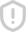### matlab动画 评分immovie 函数可以利用多帧图像创建动画，具体用法如下： 1.mov = immovie(X,map) 利用多帧索引图像X，其颜色映射为map，来创建视频，返回视频结构数组mov;关于视频结构数组的详细内容请参考getframe函数;调用implay函数播放视频。 X包含多帧索引图像，且每帧图像具有相同大小和颜色映射。X是一个大小为m*n*1*k的数组，k是图像的总帧数。（关于索引图像，可以先将这十幅图转化为索引图像，例如如果是灰度图像，则用函数[X,map]=gray2ind(I,n)转化）

...展开详情举报收藏分享MATLAB动画演示程序汇总-MATLAB 动画演示程序汇总.rar 立即下载matlab动画设计教程 立即下载matlab动画教学 立即下载matlab 动画 立即下载MATLAB动画 立即下载ajax%E7%BB%8F%E5%85%B8%E5%AE%9E%E4%BE%8B%2B%E4%BB%A3%E7%A0%81%E5%A4%A7%E5%85%A8 立即下载matlab 动画实现 立即下载MATLAB动画制作 立即下载Matlab动画制作教程 立即下载matlab 动画设计 立即下载MATLAB动画制作.doc 立即下载MATLAB动画制作及举例应用 立即下载matlab动画编程研究实例分析 立即下载MATLAB 动画生成gif图片 立即下载matlab 动画制作 课件 - 台湾清华大学 立即下载matlab动画仿真及保存为gif图片 立即下载MATLAB动画——木块在水中收到浮力上下摆动的物理过程 立即下载Matlab值得借鉴的几个动画-MATLAB动画设计.rar 立即下载ModbusTCP／RTU网关设计 立即下载lbt123456